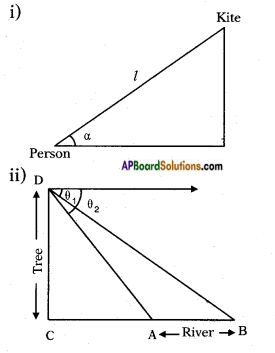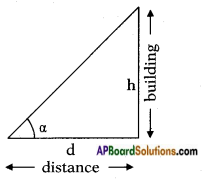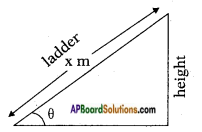# AP SSC 10th Class Maths Solutions Chapter 12 Applications of Trigonometry InText Questions

## AP State Syllabus SSC 10th Class Maths Solutions 12th Lesson Applications of Trigonometry InText Questions

AP State Board Syllabus AP SSC 10th Class Maths Textbook Solutions Chapter 12 Applications of Trigonometry InText Questions and Answers.

### 10th Class Maths 12th Lesson Applications of Trigonometry InText Questions and Answers

Do This

(Page No. 297)

Question 1.
Draw diagram for the following situations:
i) A person is flying a kite at an angle of elevation a and the length of thread from his hand to kite is ‘l’.
ii) A person observes two banks of a river at angles of depression θ1 and θ21 < θ2) from the top of a tree of height ‘h’ which is at a side of the river. The width of the river is ‘d’.In the figure
‘D’ is the position of person
CD is the height of the tree
AB is the width of the river
Angles of depression are θ1 and θ2.Think & Discuss

(Page No. 297)

Question 1.
You are observing top of your school building at an angle of elevation a from a point which is at d meter distance from foot of the building. Which trigonometric ratio would you like to consider to find the height of the building?